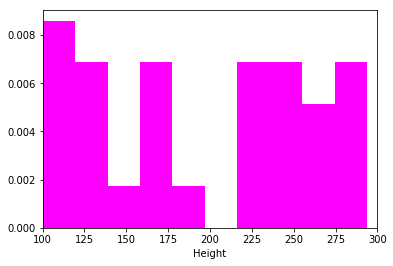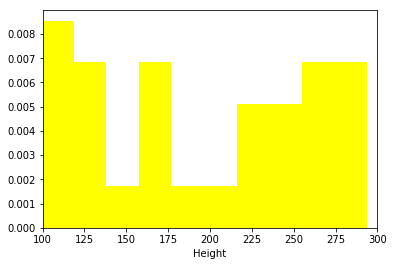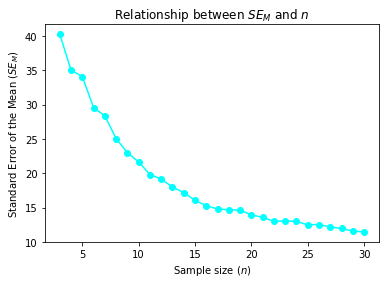# Normal Distribution Test

## SciPy stats.normaltest¶

In :
import numpy as np
from scipy import stats
import matplotlib.pyplot as plt

In :
heights_male = np.array([100, 256, 238, 116, 286, 253, 112, 165, 246, 130, 217, 269, 155,
136, 189, 235, 255, 113, 280, 222, 259, 177, 294, 290, 225, 113,
163, 137, 172, 127])

heights_female = np.array([126, 172, 137, 163, 113, 225, 290, 294, 175, 259, 220, 280, 111,
255, 235, 189, 136, 150, 269, 214, 130, 243, 165, 110, 253, 286,
116, 238, 255, 99])

print("mean heights (male): {}".format(np.mean(heights_male)))
print("mean heights (female): {}".format(np.mean(heights_female)))

mean heights (male): 197.66666666666666
mean heights (female): 196.93333333333334

In :
s, p = stats.wilcoxon(heights_female, heights_male)

if p < 0.05:
print("null hypothesis rejected, significant difference between the data-sets")
else:
print("null hypothesis accepted, no significant difference between the data-sets")

print("p value = {}".format(p))

null hypothesis accepted, no significant difference between the data-sets
p value = 0.9425801920860144

In :
plt.hist(heights_male, color="Magenta", normed=1)
plt.xlim(100,300)
plt.xlabel('Height');
plt.show()In :
plt.hist(heights_female,color="yellow", normed=1);
plt.xlim(100,300)
plt.xlabel('Height');
plt.show()In :
SEM = []

for sample_size in range(3,len(heights_male)+1):
sample = heights_male[0:sample_size]
SEM.append(sample.std() / np.sqrt(sample_size))

plt.plot(range(3,len(heights_male)+1),SEM, marker='o', color='cyan')

plt.ylabel("Standard Error of the Mean ($SE_M$)")
plt.xlabel("Sample size $(n)$")
plt.title("Relationship between $SE_M$ and $n$");In :
print(stats.normaltest(heights_male))
print(stats.normaltest(heights_female))

NormaltestResult(statistic=13.548310785013712, pvalue=0.0011429354242245898)
NormaltestResult(statistic=13.278600632632264, pvalue=0.001307942069480237)


Support this work

You can support this work by getting the e-book. This notebook will always be available for free in its online format.